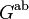# First homology group for trivial group action equals tensor product with abelianization

Suppose$G$ is a group and$A$ is an abelian group. Denote by$H_1(G,A)$ the first homology group for the trivial action of$G$ on$A$. We then have:$H_1(G,A) \cong G^{\operatorname{ab}} \otimes_{\mathbb{Z}} A$
where$G^{\operatorname{ab}}$ is the abelianization of$G$, i.e., the quotient group of$G$ by its derived subgroup, and$\otimes_{\mathbb{Z}}$ denotes the tensor product of abelian groups.